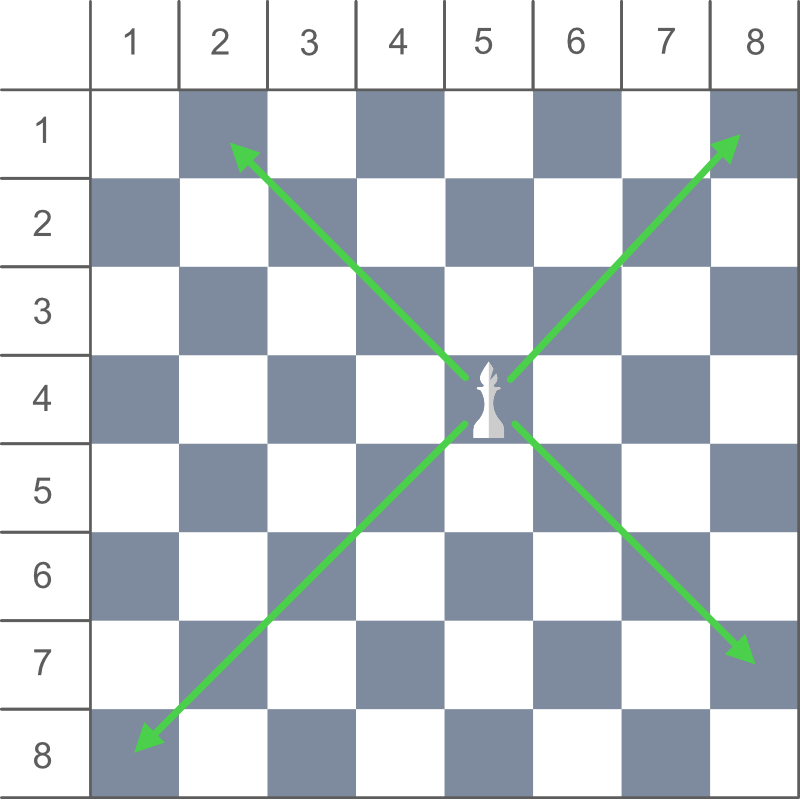# Count the total number of squares that can be visited by Bishop in one move

Given the position of a Bishop on an 8 * 8 chessboard, the task is to count the total number of squares that can be visited by the Bishop in one move. The position of the Bishop is denoted using row and column number of the chessboard.

Examples:

Input: Row = 4, Column = 4
Output: 13

Input: Row = 1, Column = 1
Output: 7

## Recommended: Please try your approach on {IDE} first, before moving on to the solution.

Approach: In the game of chess, a Bishop can only move diagonally and there is no restriction in distance for each move.So, We can also say that Bishop can move in four ways i.e. diagonally top left, top right, bottom left and bottom right from current position.

We can calculate the numbers of squares visited in each move by:

Total squares visited in Top Left move = min(r, c) – 1
Total squares visited in Top Right move = min(r, 9 – c) – 1
Total squares visited in Bottom Left move = 8 – max(r, 9 – c)
Total squares visited in Bottom Right move = 8 – max(r, c)
where, r and c are the coordinates of the current position of the Bishop on the chessboard.

Below is the implementation of the above approach:

## C++

 `// C++ implementation of above approach ` `#include ` `using` `namespace` `std; ` ` `  `// Function to return the count of ` `// total positions the Bishop ` `// can visit in a single move ` `int` `countSquares(``int` `row, ``int` `column) ` `{ ` ` `  `    ``// Count top left squares ` `    ``int` `topLeft = min(row, column) - 1; ` ` `  `    ``// Count bottom right squares ` `    ``int` `bottomRight = 8 - max(row, column); ` ` `  `    ``// Count top right squares ` `    ``int` `topRight = min(row, 9 - column) - 1; ` ` `  `    ``// Count bottom left squares ` `    ``int` `bottomLeft = 8 - max(row, 9 - column); ` ` `  `    ``// Return total count ` `    ``return` `(topLeft + topRight + bottomRight + bottomLeft); ` `} ` ` `  `// Driver code ` `int` `main() ` `{ ` ` `  `    ``// Bishop's Position ` `    ``int` `row = 4, column = 4; ` ` `  `    ``cout << countSquares(row, column); ` ` `  `    ``return` `0; ` `} `

## Java

 `// Java implementation of above approach ` `class` `GFG { ` ` `  `    ``// Function to return the count of ` `    ``// total positions the Bishop ` `    ``// can visit in a single move ` `    ``static` `int` `countSquares(``int` `row, ``int` `column) ` `    ``{ ` ` `  `        ``// Count top left squares ` `        ``int` `topLeft = Math.min(row, column) - ``1``; ` ` `  `        ``// Count bottom right squares ` `        ``int` `bottomRight = ``8` `- Math.max(row, column); ` ` `  `        ``// Count top right squares ` `        ``int` `topRight = Math.min(row, ``9` `- column) - ``1``; ` ` `  `        ``// Count bottom left squares ` `        ``int` `bottomLeft = ``8` `- Math.max(row, ``9` `- column); ` ` `  `        ``// Return total count ` `        ``return` `(topLeft + topRight + bottomRight + bottomLeft); ` `    ``} ` ` `  `    ``// Driver code ` `    ``public` `static` `void` `main(String[] args) ` `    ``{ ` ` `  `        ``// Bishop's Position ` `        ``int` `row = ``4``, column = ``4``; ` ` `  `        ``System.out.println(countSquares(row, column)); ` `    ``} ` `} `

## C#

 `// C# implementation of above approach ` `using` `System; ` `class` `GFG { ` ` `  `    ``// Function to return the count of ` `    ``// total positions the Bishop ` `    ``// can visit in a single move ` `    ``static` `int` `countSquares(``int` `row, ``int` `column) ` `    ``{ ` ` `  `        ``// Count top left squares ` `        ``int` `topLeft = Math.Min(row, column) - 1; ` ` `  `        ``// Count bottom right squares ` `        ``int` `bottomRight = 8 - Math.Max(row, column); ` ` `  `        ``// Count top right squares ` `        ``int` `topRight = Math.Min(row, 9 - column) - 1; ` ` `  `        ``// Count bottom left squares ` `        ``int` `bottomLeft = 8 - Math.Max(row, 9 - column); ` ` `  `        ``// Return total count ` `        ``return` `(topLeft + topRight + bottomRight + bottomLeft); ` `    ``} ` ` `  `    ``// Driver code ` `    ``public` `static` `void` `Main() ` `    ``{ ` ` `  `        ``// Bishop's Position ` `        ``int` `row = 4, column = 4; ` ` `  `        ``Console.WriteLine(countSquares(row, column)); ` `    ``} ` `} `

## Python3

 `# Python3 implementation of above approach ` ` `  `# Function to return the count of  ` `# total positions the Bishop  ` `# can visit in a single move ` `def` `countSquares(row, column): ` `     `  `    ``# Count top left squares ` `    ``topLeft ``=` `min``(row, column) ``-` `1` `     `  `    ``# Count bottom right squares ` `    ``bottomRight ``=` `8` `-` `max``(row, column) ` `     `  `    ``# Count top right squares ` `    ``topRight ``=` `min``(row, ``9``-``column) ``-``1` `     `  `    ``# Count bottom left squares ` `    ``bottomLeft ``=` `8` `-` `max``(row, ``9``-``column)  ` ` `  `     `  `    ``# Return total count ` `    ``return` `(topLeft ``+` `topRight ``+` `bottomRight ``+` `bottomLeft) ` ` `  `# Driver code ` ` `  `# Bishop's Position  ` `row ``=` `4` `column ``=` `4` ` `  `print``(countSquares(row, column)) `

## PHP

 ` `

Output:

```13
```My Personal Notes arrow_drop_upCheck out this Author's contributed articles.

If you like GeeksforGeeks and would like to contribute, you can also write an article using contribute.geeksforgeeks.org or mail your article to contribute@geeksforgeeks.org. See your article appearing on the GeeksforGeeks main page and help other Geeks.

Please Improve this article if you find anything incorrect by clicking on the "Improve Article" button below.

Improved By : jit_t